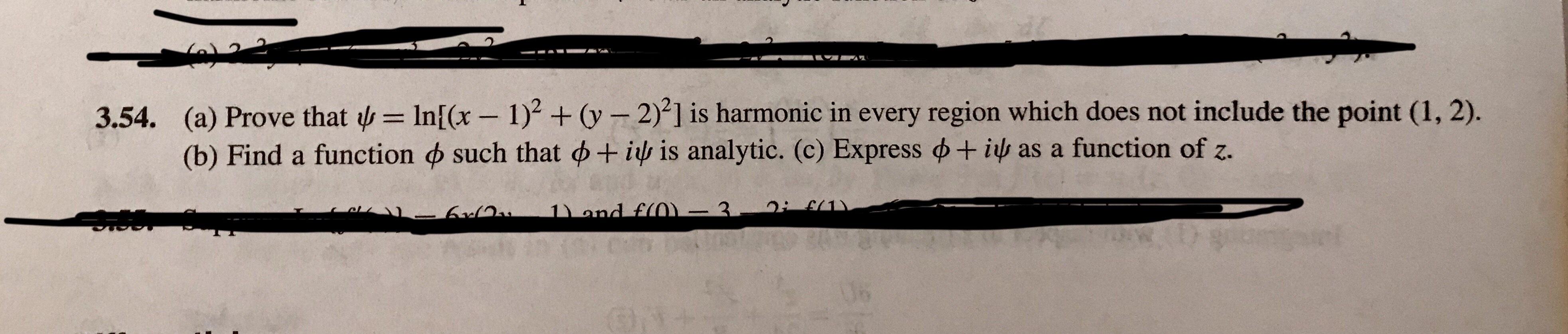1 )2 + ơ-2)2] is harmonic in every region which does not include the point (1,2).(a) Prove that ψ = Inf(x-(b) Find a function ф such that ф + ir is analytic. (c) Express ф+3.54.as a function of z.

Question

Question attached. Z is a complex variable, z=x+iyhelp_outlineImage Transcriptionclose1 )2 + ơ-2)2] is harmonic in every region which does not include the point (1,2). (a) Prove that ψ = Inf(x- (b) Find a function ф such that ф + ir is analytic. (c) Express ф+ 3.54. as a function of z. fullscreen
Step 1

To verify the Laplace equation (harmonicity condition ) for the given function and to find its harmonic conjugate

Step 2

A real function V(x,y) is said to be harmonic if V satisfies the Laplace equation: (here subscripts denote partial derivatives)

Vxx+Vyy=0

Step 3

Let us verify that the given fun...

Want to see the full answer?

See Solution

Want to see this answer and more?

Our solutions are written by experts, many with advanced degrees, and available 24/7

See Solution
Tagged in

Math